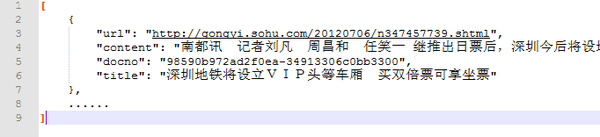# 搜狐新闻文本分类：机器学习大乱斗

#### 目标

• 从头开始实践中文短文本分类，记录一下实验流程与遇到的
• 运用多种机器学习（深度学习 + 传统机器学习）方法比较短文本分类处理过程与结果差别

#### 工具

• 深度学习：keras
• 传统机器学习：sklearn

#### 参与比较的机器学习方法

1. CNN 、 CNN + word2vec
2. LSTM 、 LSTM + word2vec
3. MLP（多层感知机）
4. 朴素贝叶斯
5. KNN
6. SVM
7. SVM + word2vec 、SVM + doc2vec

#### 实验结论

• 引入预训练的 word2vec 模型会给训练带来好处，具体来说：（1）间接引入外部训练数据，防止过拟合；（2）减少需要训练的参数个数，提高训练效率
• LSTM 需要训练的参数个数远小于 CNN，但训练时间大于 CNN。CNN 在分类问题的表现上一直很好，无论是图像还是文本；而想让 LSTM 优势得到发挥，首先让训练数据量得到保证
• 将单词在 word2vec 中的词向量加和求平均获得整个句子的语义向量的方法看似 naive 有时真挺奏效，当然仅限于短句子，长度 100 以内应该可以
• 机器学习方法万千，具体选择用什么样的方法还是要取决于数据集的规模以及问题本身的复杂度，对于复杂程度一般的问题，看似简单的方法有可能是坠吼地

# 0 数据预处理UltraEdit 就是好就是秒就是呱呱叫

11个类分别是

jieba真是好真是秒真是呱呱叫

# 1 CNN

#### 不使用预训练 word2vec 模型的 CNN：

``````MAX_SEQUENCE_LENGTH = 100 # 每条新闻最大长度
EMBEDDING_DIM = 200 # 词向量空间维度
VALIDATION_SPLIT = 0.16 # 验证集比例
TEST_SPLIT = 0.2 # 测试集比例
``````

``````from keras.preprocessing.text import Tokenizer
from keras.utils import to_categorical
import numpy as np

tokenizer = Tokenizer()
tokenizer.fit_on_texts(all_texts)
sequences = tokenizer.texts_to_sequences(all_texts)
word_index = tokenizer.word_index
print('Found %s unique tokens.' % len(word_index))
labels = to_categorical(np.asarray(all_labels))
print('Shape of data tensor:', data.shape)
print('Shape of label tensor:', labels.shape)
``````

``````p1 = int(len(data)*(1-VALIDATION_SPLIT-TEST_SPLIT))
p2 = int(len(data)*(1-TEST_SPLIT))
x_train = data[:p1]
y_train = labels[:p1]
x_val = data[p1:p2]
y_val = labels[p1:p2]
x_test = data[p2:]
y_test = labels[p2:]
print 'train docs: '+str(len(x_train))
print 'val docs: '+str(len(x_val))
print 'test docs: '+str(len(x_test))

``````

``````from keras.layers import Dense, Input, Flatten, Dropout
from keras.layers import Conv1D, MaxPooling1D, Embedding
from keras.models import Sequential

model = Sequential()
model.summary()
``````

：这里只使用了 1 层卷积层，为什么不多加几层？
：新闻长度只有100个单词，即特征只有100维，1 层卷积加池化后特征已经缩减为 32 维，再加卷积可能就卷没了。 2 层 3 层也做过，效果都不好
：为什么只训练 2 轮
：因为是遍历训练数据不是随机抽取，1 轮训练已经接近饱和，实验表明第 2 轮训练基本没什么提升
：为什么卷积核为什么选择 250 个？
：因为开心

#### 基于预训练的 word2vec 的 CNN ：

keras示例程序 pretrained_word_embeddings.py 代码地址
https://github.com/fchollet/keras/blob/master/examples/pretrained_word_embeddings.py

``````VECTOR_DIR = 'wiki.zh.vector.bin' # 词向量模型文件

from keras.utils import plot_model
from keras.layers import Embedding
import gensim

embedding_matrix = np.zeros((len(word_index) + 1, EMBEDDING_DIM))
for word, i in word_index.items():
if unicode(word) in w2v_model:
embedding_matrix[i] = np.asarray(w2v_model[unicode(word)],
dtype='float32')
embedding_layer = Embedding(len(word_index) + 1,
EMBEDDING_DIM,
weights=[embedding_matrix],
input_length=MAX_SEQUENCE_LENGTH,
trainable=False)
``````

``````from keras.layers import Dense, Input, Flatten, Dropout
from keras.layers import Conv1D, MaxPooling1D, Embedding
from keras.models import Sequential

model = Sequential()
model.summary()
#plot_model(model, to_file='model.png',show_shapes=True)
model.compile(loss='categorical_crossentropy',
optimizer='rmsprop',
metrics=['acc'])
model.fit(x_train, y_train, validation_data=(x_val, y_val), epochs=2, batch_size=128)
model.save('word_vector_cnn.h5')
print model.evaluate(x_test, y_test)
``````

# 2 LSTM

#### 不使用预训练的 word2vec 模型的 LSTM：

``````from keras.layers import Dense, Input, Flatten, Dropout
from keras.layers import LSTM, Embedding
from keras.models import Sequential

model = Sequential()
input_length=MAX_SEQUENCE_LENGTH))
model.summary()
``````

#### 基于预训练的 word2vec 模型：

``````from keras.layers import Dense, Input, Flatten, Dropout
from keras.layers import LSTM, Embedding
from keras.models import Sequential

model = Sequential()
model.summary()
``````

# 3 MLP（多层感知机）

keras 示例程序 reuters_mlp.py 代码地址
https://github.com/fchollet/keras/blob/master/examples/reuters_mlp.py

MLP 是一个结构上很简单很 naive 的神经网络。数据的处理流程也跟上面两个实验差不多，不过不再将每条新闻处理成 100 x 200 的 2 维向量，而是成为长度 65604 的 1 维向量。65604 代表数据集中所有出现的 65604 个单词，数据的值用 tf-idf 值填充，整个文档集成为一个用 17600 x 65604 个 tf-idf 值填充的矩阵，第 i 行 j 列的值表征了第 j 个单词在第 i 个文档中的的 tf-idf值（当然这里也可以不用 tf-idf 值，而只是使用 0/1 值填充， 0/1 代表第 j 个单词在第 i 个文档中是否出现，但是实验显示用 tf-idf 的效果更好）

``````tokenizer = Tokenizer()
tokenizer.fit_on_texts(all_texts)
sequences = tokenizer.texts_to_sequences(all_texts)
word_index = tokenizer.word_index
print('Found %s unique tokens.' % len(word_index))
data = tokenizer.sequences_to_matrix(sequences, mode='tfidf')
labels = to_categorical(np.asarray(all_labels))
``````

``````from keras.layers import Dense, Dropout
from keras.models import Sequential

model = Sequential()
model.summary()
``````

# 4 朴素贝叶斯

``````from sklearn.feature_extraction.text import CountVectorizer, TfidfTransformer
count_v0= CountVectorizer();
counts_all = count_v0.fit_transform(all_text);
count_v1= CountVectorizer(vocabulary=count_v0.vocabulary_);
counts_train = count_v1.fit_transform(train_texts);
print "the shape of train is "+repr(counts_train.shape)
count_v2 = CountVectorizer(vocabulary=count_v0.vocabulary_);
counts_test = count_v2.fit_transform(test_texts);
print "the shape of test is "+repr(counts_test.shape)

tfidftransformer = TfidfTransformer();
train_data = tfidftransformer.fit(counts_train).transform(counts_train);
test_data = tfidftransformer.fit(counts_test).transform(counts_test);
``````

``````from sklearn.naive_bayes import MultinomialNB
from sklearn import metrics
clf = MultinomialNB(alpha = 0.01)
clf.fit(x_train, y_train);
preds = clf.predict(x_test);
num = 0
preds = preds.tolist()
for i,pred in enumerate(preds):
if int(pred) == int(y_test[i]):
num += 1
print 'precision_score:' + str(float(num) / len(preds))
``````

# 5 KNN

``````from sklearn.neighbors import KNeighborsClassifier

for x in range(1,15):
knnclf = KNeighborsClassifier(n_neighbors=x)
knnclf.fit(x_train,y_train)
preds = knnclf.predict(x_test);
num = 0
preds = preds.tolist()
for i,pred in enumerate(preds):
if int(pred) == int(y_test[i]):
num += 1
print 'K= '+str(x)+', precision_score:' + str(float(num) / len(preds))
``````

K=11时，准确度 0.31961147，非常低，KNN 方法还是不太适合做此类问题

# 6 SVM

``````from sklearn.svm import SVC
svclf = SVC(kernel = 'linear')
svclf.fit(x_train,y_train)
preds = svclf.predict(x_test);
num = 0
preds = preds.tolist()
for i,pred in enumerate(preds):
if int(pred) == int(y_test[i]):
num += 1
print 'precision_score:' + str(float(num) / len(preds))
``````

# 7 SVM + word2vec 与 doc2vec

#### svm + word2vec：

``````import gensim
import numpy as np
x_train = []
x_test = []
for train_doc in train_docs:
words = train_doc.split(' ')
vector = np.zeros(EMBEDDING_DIM)
word_num = 0
for word in words:
if unicode(word) in w2v_model:
vector += w2v_model[unicode(word)]
word_num += 1
if word_num > 0:
vector = vector/word_num
x_train.append(vector)
for test_doc in test_docs:
words = test_doc.split(' ')
vector = np.zeros(EMBEDDING_DIM)
word_num = 0
for word in words:
if unicode(word) in w2v_model:
vector += w2v_model[unicode(word)]
word_num += 1
if word_num > 0:
vector = vector/word_num
x_test.append(vector)
``````

#### svm + doc2vec：

``````import gensim
sentences = gensim.models.doc2vec.TaggedLineDocument('all_contents.txt')
model = gensim.models.Doc2Vec(sentences, size=200, window=5, min_count=5)
model.save('doc2vec.model')
print 'num of docs: ' + str(len(model.docvecs))
``````

all_contents.txt 里是包括训练文档与测试文档在内的所有数据，同样每行 1 条新闻，由若干个词组成，词之间用空格隔开，先使用 gensim 的 TaggedLineDocument 函数预处理下，然后直接使用 Doc2Vec 函数开始训练，训练过程很快（可能因为数据少）。然后这所有 21924 篇新闻就变成了 21924 个长度 200 的向量，取出前 17600 个给 SVM 做分类训练，后 4324 个测试，代码和结果如下：

``````import gensim
x_train = []
x_test = []
y_train = train_labels
y_test = test_labels
for idx, docvec in enumerate(model.docvecs):
if idx < 17600:
x_train.append(docvec)
else:
x_test.append(docvec)
print 'train doc shape: '+str(len(x_train))+' , '+str(len(x_train))
print 'test doc shape: '+str(len(x_test))+' , '+str(len(x_test))

from sklearn.svm import SVC
svclf = SVC(kernel = 'rbf')
svclf.fit(x_train,y_train)
preds = svclf.predict(x_test);
num = 0
preds = preds.tolist()
for i,pred in enumerate(preds):
if int(pred) == int(y_test[i]):
num += 1
print 'precision_score:' + str(float(num) / len(preds))
``````

# 总结

• 引入预训练的 word2vec 模型会给训练带来好处，具体来说：（1）间接引入外部训练数据，防止过拟合；（2）减少需要训练的参数个数，提高训练效率
• LSTM 需要训练的参数个数远小于 CNN，但训练时间大于 CNN。CNN 在分类问题的表现上一直很好，无论是图像还是文本；而想让 LSTM 优势得到发挥，首先让训练数据量得到保证
• 将单词在 word2vec 中的词向量加和求平均获得整个句子的语义向量的方法看似 naive 有时真挺奏效，当然仅限于短句子，长度 100 以内应该可以
• 机器学习方法万千，具体选择用什么样的方法还是要取决于数据集的规模以及问题本身的复杂度，对于复杂程度一般的问题，看似简单的方法有可能是坠吼地